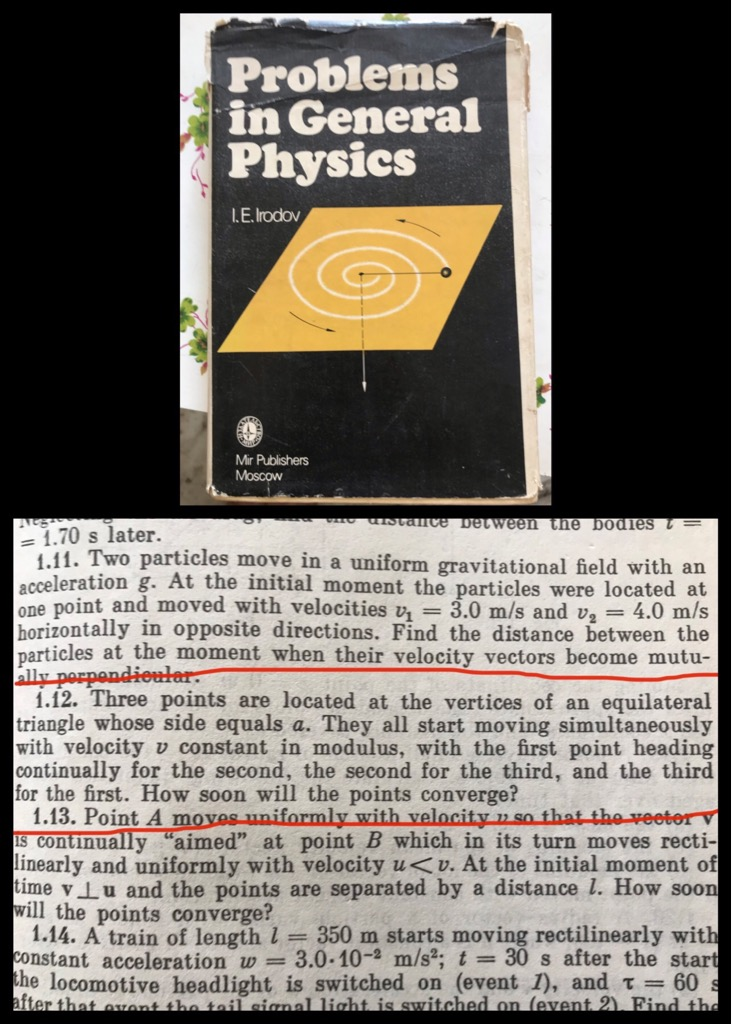3 November 2019

# A hard puzzle from India!

Tuesday afternoon, I was all by myself. Both dad and mom were sleeping. Not knowing anything better, I went around going thru the books that still adorn the book shelves in my parents’ house. These are books from my school days. They have kept them still. Most of them are disintegrating – but they are still there. After going thru some of the books that I had studied in high school, I chanced upon this book I still remember for very hard math/physics problems. Written by Irodov, it was a book I had bought from Kolkata Book Fair in 1984.

I glanced thru a few pages. Sure enough, they are as hard as I remember them. There was one that caught my attention as a very interesting one. You can see the one in the picture that I have circled with the red line.

This is a very tough problem until you hit the solution and realize how elegant the solution is.

Here is a version of the problem:

There is an equilateral triangle – each of side length “a”. There are three ants at the three corners. Let’s call the ants A,B and C. At the same moment the three ants start moving at exactly the same speed. Ant A keeps moving towards wherever Ant B is. Ant B keeps moving towards wherever Ant C is. And Ant C keeping moving towards wherever Ant A is. The question is : eventually when they meet, how much distance would each ant have traveled?

Now realize that for every ant, the target ant is moving continuously. So, every ant is continually changing its direction. It is not as simple as an ant goes from one vertex to the other. That is what makes the problem hard.

After thinking about the problem for some time – and not getting anywhere – I posed it to my brother when I met him two days later. Together we spent about an hour in our drive to Kalyani from Kolkata discussing the problem. Eventually, we reached Kalyani and asked mom for some paper and pen. Another half an hour later, we did manage to solve the problem. Excitedly, we pulled out the Irodov book again from the shelf to see if the answer it had given matched ours. It did!!!

This was only Chapter 1 of the book and the 12th problem in it!! That chapter alone had another hundred plus problems. And then there were many more chapters!!

Man, I am way past my prime when it comes to ability to solve these kind of problems.

Anyways, see if this excites you to give it a crack.

If you get it, try the same problem with a square of side “a” instead of an equilateral triangle.Posted November 3, 2019 by Rajib Roy in category "Puzzles", "Vacations

1.By rajibroy (Post author) on

Let’s start with the equilateral triangle ABC. Let’s say each ant is moving at the velocity of v.
Since the problem is symmetric for the three ants, they have to meet at the centroid of the triangle.

When Ant A has moved (dx) (delta x = infinitesimally small distance), so has Ant B and Ant C. Notice that they are again in an equilateral triangle and they will meet at the same centroid point as before.

When Ant A moves the next (dx) (at a slightly different direction than before), they are again in an equilateral triangle.

Further note, that every point, the ants are moving with speed |v| (modulus of velocity v). And the direction is always towards the centroid. The component at every point of that velocity towards the centroid is v*cos30 degree (the angle between the equilateral side and the straight line to the centroid from the vertex). cos 30 being root(3)/2 , the component is v*root(3)/2

In essence, each of three ants is moving towards the centroid at a component velocity of v*root(3)/2 in a fashion so as to maintain an equilateral triangle at all times. The displacement from original time to the meeting point (centroid) is two thirds the length of the median which is 2/3 of root(3)*a/2 = a/root(3)

The time taken = displacement / velocity (component in that direction) = [a/root(3)] / [v*root(3)/2] = 2a/3v

The distance traveled = speed * time = 2a/3v * |v| = 2a/3 (2/3rds the size of a side of the equilateral triangle.

For the square, the velocity component is v*cos45 degree = v / root(2). The displacement is half the diagonal which is 1/2 of a*root(2) = a / root (2)

The time is [a/root(2)]/(v/root(2)] = a/v.

The distance is a/v * v = a (the same length as the side of the square)

2.By Jared Bronski on

This is a nice puzzle. I have seen it before but always did it by solving the differential equation and finding the curve. Your post made me look for a more elementary and more elegant solution, and I came up with basically the same solution that you and your brother found. If there are N ants on a regular N-gon with side length d the time until they reach the centroid is d/(v(1- cos 2Pi/n)) and the distance traveled by each ant is d/(1-cos 2Pi/n). If there are two ants on a line segment they travel d/2, if there are three on an equilateral triangle 2d/3, and so on. As n tends to infinity the poor ants travel further and further.

This site uses Akismet to reduce spam. Learn how your comment data is processed.